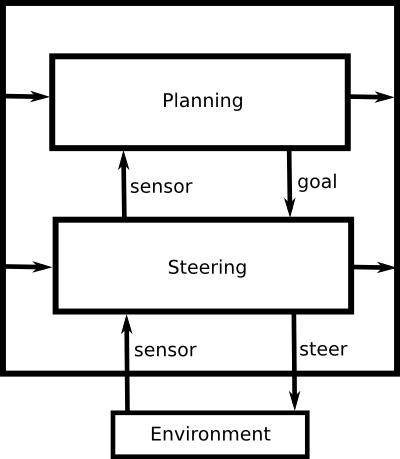Practice Exercise 2.A
Hierarchical Control

## 2: Learning Goals

• Design a simple hierarchical controller
• Implement a component of a hierarchical controller
• Predict the behavior of a hierarchical controller in a simple domain.

## 3: Directed Questions

1. If you were the designer of a hierarchical controller, which layer would you assign to have the fastest update frequency? [solution]

2. What are the three types of outputs for a given layer in a hierarchical controller? [solution]

## 4: Exercise: Tortoises

Some of the world's first robots were designed by Grey Walter. These robots were referred to as "tortoises" due to their shape and movement style (Walter referred to the robots as "Machina speculatrix"). Braitenberg vehicles were inspired by Walter's tortoises. The tortoises had a light sensor and powered wheels. In this exercise we will develop a hierarchical controller to control the tortoises.

The lowest layer of the hierarchical controller has a single input and a single output. The input is the amount of light received by the light sensor. The output is a binary decision on whether the robot should curve to the left or the right. The robot always moves forward, so the only action it can make is to either steer left or steer right.

If we want the robot to be able to follow the gradient of a light source, what information does it need to remember between time steps? How can this information be stored in a hierarchical controller? [solution]

Consider the following two layer robot controller:In order to design the robot controller, we need to decide on some task we want the tortoise to accomplish. Let's say that it should start by following the light gradient to move closer to a light source. Once it reaches a certain light threshold, it should then turn around and start following the gradient away from the light source. Once it reaches a certain darkness threshold, it should then start over and start moving towards the light source again.

In a programming language of your choice, implement the top level of the robot controller hierarchy. [solution]

In a programming language of your choice, implement the bottom level of the robot controller hierarchy. [solution]

If the tortoise had two light sensors instead of one, in general could it be better at following the light gradient? If so, provide a brief description of an algorithm it could use. [solution]

Let's change the tortoise's task to always follow the light gradient in order to try to move towards a light source. Let's assume that you build a tortoise robot and install robot controller software on it which always follows the light gradient. Now you want to perform experiments on the robot in your room. You have a lamp that can act as a light source and the tortoise can move across the floor of your room.

If there was a box between the tortoise and the lamp, how might it react to the box? [solution]

Can you think of an arrangement of obstacles that would make the tortoise move away from the lamp? [solution]

Design a trap that would prevent your robot controller from ever reaching the lamp. There must be a valid path from the robot's starting position to the lamp (which your robot controller will not find). [solution]

## 5: Learning Goals Revisited

• Design a simple hierarchical controller
• Implement a component of a hierarchical controller
• Predict the behavior of a hierarchical controller in a simple domain.